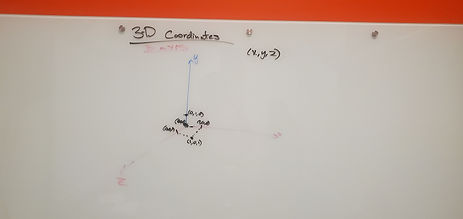## Mr. Jake

### Target 1​

###### Lesson Type:

New

Algebra

:

Function

Graphically represent function equations in the coordinate plane.

###### 1:

Identify the different representations of a function on a graph.

###### 2:

Interpret the relationship between x and y values.

###### 3:

Justify whether a graphic representation is a function.

HS

###### Vocabulary:

Exponential, function, parabola

Activities:

Plotted different exponential functions

Discussed why exponential functions look the way they do.### Home Exploration

###### Guiding Questions:## Absent Students:

### Target 2

:

###### 1:

Apply 2D understandings of graphing (x- and y-axis) to 3D representations on the coordinate plane with the introduction of the z-axis.

HS

###### Vocabulary:

Z-Axis, 3D, Model

Activities:

Created a 3D coordinate plane to determine the coordinates of 3D figures

Explored the z axis using understanding of x and y axis### Home Exploration

###### Guiding Questions:### Target 3

:

###### Vocabulary:

Activities:### Home Exploration